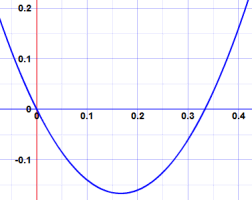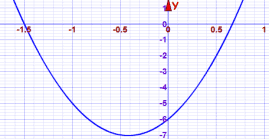A Quadratic Equation in Standard Form
(a, b, and c can have any value, except that a can't be 0.)

To "Factor" (or "Factorise" in the UK) a Quadratic is to:

find what to multiply to get the Quadratic

It is called "Factoring" because we find the factors (a factor is something we multiply by)

### Example:

Multiplying (x+4) and (x−1) together (called Expanding) gets x2 + 3x − 4 :So (x+4) and (x−1) are factors of x2 + 3x − 4

Just to be sure, let us check:

(x+4)(x−1)  = x(x−1) + 4(x−1)
= x2 − x + 4x − 4
= x2 + 3x − 4Yes, (x+4) and (x−1) are definitely factors of x2 + 3x − 4

Did you see that Expanding and Factoring are opposites?Expanding is usually easy, but Factoring can often be tricky.It is like trying to find which ingredients
went into a cake to make it so delicious.
It can be hard to figure out!

So let us try an example where we don't know the factors yet:

## Common Factor

First check if there any common factors.

### Example: what are the factors of 6x2 − 2x = 0 ?

6 and 2 have a common factor of 2:

2(3x2 − x) = 0

And x2 and x have a common factor of x:

2x(3x − 1) = 0

And we have done it! The factors are 2x and 3x − 1,

We can now also find the roots (where it equals zero):

• 2x is 0 when x = 0
• 3x − 1 is zero when x = 13

And this is the graph (see how it is zero at x=0 and x=13):But it is not always that easy ...

## Guess and Check

Maybe we can guess an answer?

### Example: what are the factors of 2x2 + 7x + 3 ?

No common factors.

Let us try to guess an answer, and then check if we are right ... we might get lucky!

We could guess (2x+3)(x+1):

(2x+3)(x+1) = 2x2 + 2x + 3x + 3
= 2x2 + 5x + 3
(WRONG)

(2x+7)(x−1) = 2x2 − 2x + 7x − 7
= 2x2 + 5x − 7
(WRONG AGAIN)

(2x+9)(x−1) = 2x2 − 2x + 9x − 9
= 2x2 + 7x − 9
(WRONG AGAIN)

Oh No! We could be guessing for a long time before we get lucky.

That is not a very good method. So let us try something else.

## A Method For Simple Cases

Luckily there is a method that works in simple cases.

With the quadratic equation in this form:Step 1: Find two numbers that multiply to give ac (in other words a times c), and add to give b.

Example: 2x2 + 7x + 3

ac is 2×3 = 6 and b is 7

So we want two numbers that multiply together to make 6, and add up to 7

In fact 6 and 1 do that (6×1=6, and 6+1=7)

How do we find 6 and 1?

It helps to list the factors of ac=6, and then try adding some to get b=7.

Factors of 6 include 1, 2, 3 and 6.

Aha! 1 and 6 add to 7, and 6×1=6.

Step 2: Rewrite the middle with those numbers:

Rewrite 7x with 6x and 1x:

2x2 + 6x + x + 3

Step 3: Factor the first two and last two terms separately:

The first two terms 2x2 + 6x factor into 2x(x+3)

The last two terms x+3 don't actually change in this case

So we get:

2x(x+3) + (x+3)

Step 4: If we've done this correctly, our two new terms should have a clearly visible common factor.

In this case we can see that (x+3) is common to both terms, so we can go:

Which is:2x(x+3) + 1(x+3)
And so:(2x+1)(x+3)

Done!

Check: (2x+1)(x+3) = 2x2 + 6x + x + 3 = 2x2 + 7x + 3 (Yes)

Much better than guessing!

Let's see Steps 1 to 4 again, in one go:

 2x2 + 7x + 3 2x2 + 6x + x + 3 2x(x+3) + (x+3) 2x(x+3) + 1(x+3) (2x+1)(x+3)

### Example: 6x2 + 5x − 6

Step 1: ac is 6×(−6) = −36, and b is 5

List the positive factors of ac = −36: 1, 2, 3, 4, 6, 9, 12, 18, 36

One of the numbers has to be negative to make −36, so by playing with a few different numbers I find that −4 and 9 work nicely:

−4×9 = −36 and −4+9 = 5

Step 2: Rewrite 5x with −4x and 9x:

6x2 − 4x + 9x − 6

Step 3: Factor first two and last two:

2x(3x − 2) + 3(3x − 2)

Step 4: Common Factor is (3x − 2):

(2x+3)(3x − 2)

Check: (2x+3)(3x − 2) = 6x2 − 4x + 9x − 6 = 6x2 + 5x − 6 (Yes)

### Finding Those Numbers

The hardest part is finding two numbers that multiply to give ac, and add to give b.

It is partly guesswork, and it helps to list out all the factors.

### Example: ac = −120 and b = 7

What two numbers multiply to −120 and add to 7 ?

The factors of 120 are (plus and minus):

1, 2, 3, 4, 5, 6, 8, 10, 12, 15, 20, 24, 30, 40, 60, and 120

We can try pairs of factors (start near the middle!) and see if they add to 7:

• −10 x 12 = −120, and −10+12 = 2 (no)
• −8 x 15 = −120 and −8+15 = 7 (YES!)

## Why Factor?

Well, one of the big benefits of factoring is that we can find the roots of the quadratic equation (where the equation is zero).

All we need to do (after factoring) is find where each of the two factors becomes zero

### Example: what are the roots (zeros) of 6x2 + 5x − 6 ?

We already know (from above) the factors are

(2x + 3)(3x − 2)

And we can figure out that

(2x + 3) is zero when x = −3/2

and

(3x − 2) is zero when x = 2/3

So the roots of 6x2 + 5x − 6 are:

−3/2 and 2/3

Here is a plot of 6x2 + 5x − 6, can you see where it equals zero?And we can also check it using a bit of arithmetic:

At x = -3/2: 6(-3/2)2 + 5(-3/2) - 6 = 6×(9/4) - 15/2 - 6 = 54/4 - 15/2 - 6 = 6-6 = 0

At x = 2/3: 6(2/3)2 + 5(2/3) - 6 = 6×(4/9) + 10/3 - 6 = 24/9 + 10/3 - 6 = 6-6 = 0

## Graphing

We can also try graphing the quadratic equation. Seeing where it equals zero can give us clues.

### Example: (continued)

Starting with 6x2 + 5x − 6 and just this plot:The roots are around x = −1.5 and x = +0.67, so we can guess the roots are:

−3/2 and 2/3

Which can help us work out the factors 2x + 3 and 3x − 2

Always check though! The graph value of +0.67 might not really be 2/3

## The General Solution

There is also a general solution (useful when the above method fails), which uses the quadratic formula:Use that formula to get the two answers x+ and x (one is for the "+" case, and the other is for the "−" case in the "±"), and we get this factoring:

a(x − x+)(x − x)

Let us use the previous example to see how that works:

### Example: what are the roots of 6x2 + 5x − 6 ?

Substitute a=6, b=5 and c=−6 into the formula:

x = −b ± √(b2 − 4ac)2a

= −5 ± √(52 − 4×6×(−6))2×6

= −5 ± √(25 + 144)12

= −5 ± √16912

= −5 ± 1312

So the two roots are:

x+ = (−5 + 13) / 12 = 8/12 = 2/3,

x = (−5 13) / 12 = −18/12 = −3/2

(Notice that we get the same answer as when we did the factoring earlier.)

Now put those values into a(x − x+)(x − x):

6(x − 2/3)(x + 3/2)

We can rearrange that a little to simplify it:

3(x − 2/3) × 2(x + 3/2) = (3x − 2)(2x + 3)

And we get the same factors as we did before.